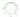## 二次对合

Sat, 7th December 2019Edit on Github二次对合几何变换

# 摘要

2011年1月mathe提出了一个问题

# 二次对合变换

## 简单的二重迭代

$C, C_1$是平面上的两条圆锥曲线. $C$（上的点列）是$C_1$作用对象，$C_1$是变换曲线。
$C_1$上的动点$Q$$C$的两条切线得切点$P$$R$，那么$P\rightarrow R$就确定了$C$上点列的一个变换，且称之为$C$关于$C_1$的一个二次对合变换（不知道数学上是否已经有这个变换的定义，需要注意的是二次对合变换不是对合变换）。

$PR$$Q$关于$C$的极线，其直线方程为$2x_0x-y-y_0=0$.
$PR$$C$交于$(x_1,y_1),(x_2,y_2)$两点，也就是方程$x^2-2x_0x+y_0=0$的两根是$x_1,x_2$, 由韦达定理可得$x_1+x_2=2x_0,x_1x_2=y_0$,

$(\frac{x_1+x_2}2,x_1x_2)$代入方程得
$A(x_1+x_2)^2+B(x_1+x_2)x_1x_2+C(x_1x_2)^2+D(x_1+x_2)+E(x_1x_2)+F=0$ (1)

$\begin{bmatrix}x_1&x_1^2&1\end{bmatrix}\begin{bmatrix}2A+E&B&D\\B&C&A\\D&A&F\end{bmatrix}\begin{bmatrix}x_2\\x_2^2\\1\end{bmatrix}=0$ (2)

$x_{2_1}\rightarrow x_{2_2}$$x_{2_1}\rightarrow x_1$$x_1\rightarrow x_{2_2}$的复合，故得二次对合变换的平方还是二次对合变换。

(2)中矩阵我们还可以看成二次曲线$C_3$:
$(x,y,z)\begin{bmatrix}2A+E&B&D\\B&C&A\\D&A&F\end{bmatrix} \begin{bmatrix}x\\y\\z\end{bmatrix}=0$

## 二次对合变换的等价定义

i)给定圆锥曲线$C$,以及另外一个圆锥曲线$C_1$,对于$C$上任意一点$P_1$,过$P_1$$C_1$的任意一条切线交$C$$P_2$,同样过$P_2$$C_1$的另外一条切线交$C$$P_3$,...

ii)对偶的，给定圆锥曲线$C$,以及另外一个圆锥曲线$C_1$,对于$C$上任意一点$P_1$,$P_1$点的$C$的切线交于$C_1$于一点，而过着一点可以做$C_1$的另外一条切线，切点为$P_2$, 同样$P_2$的这条切线交$C_1$于另外一点，而过这一点$C$的另外一条切线的切点为$P_3$,...

iii)给定圆锥曲线$C$$C_1$,对于$C$上任意一点$P_1$,$P_1$关于$C_1$的极线和$C$的一个交点定义为$P_2$,然后$P_2$关于$C_1$的极线（必然过$P_1$）和$C$的另外一个交点为$P_3$,$P_3$关于$C_1$的极线和$C$的另外一个交点为$P_4$,.... 依次类推，我们可以得到一个序列$P_1,P_2,P_3,\dots,P_n,\dots$

iv)对偶于iii),给定圆锥曲线$C$$C_1$,对于$C$上任意一点$P_1$,$P_1$处切线关于$C_1$的极点可以向$C$引任意一切线，切点定义为$P_2$,然后$P_2$处切线关于$C_1$的极点向$C$做另外一条切线（其中一条必然切于$P_1$)切$C$$P_3$,.... 依次类推，我们可以得到一个序列$P_1,P_2,P_3,\dots,P_n,\dots$。 我们称$P_1$关于这个二次对合变换的像是$P_2$,$P_2$关于这个二次对合变换的像是$P_3$,... 而这个序列是对应的一个二次对合变换序列。

## 二次对合变换的驻点

$P_2,P_1,P_1,P_2,\dots$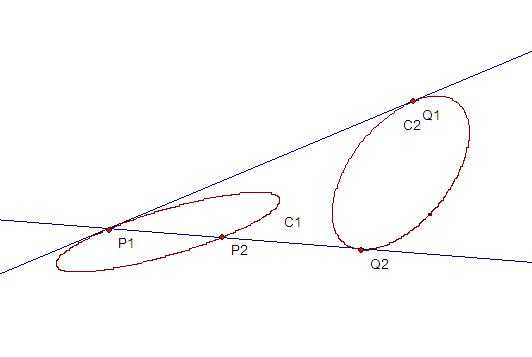## 二次对合变换的反射点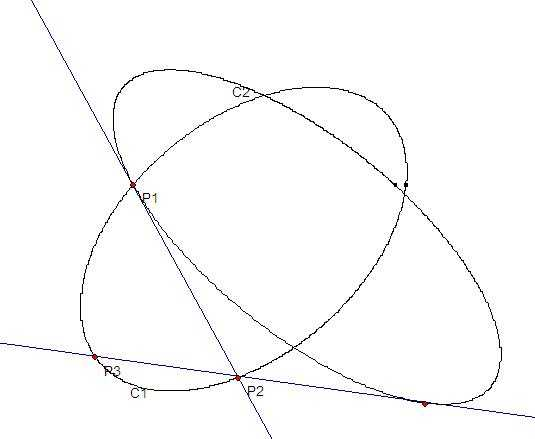$P_1$是两曲线一个交点，过$P_1$分别作出变换的后面两个点$P_2,P_3$, 如果现在我们反过来从$P_3$开始序列，并且将第二个点选择为$P_2$,于是继续变换下去我们得到序列
$P_3,P_2,P_1,P_2,P_3,\dots$,

## 二次对合变换的复合变换问题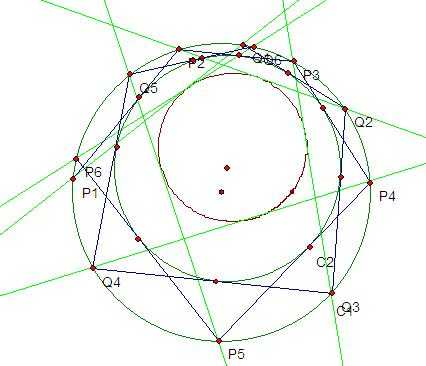（同样对偶的设$P_1$点的切线和$Q_1$点的切线交于$R_1,P_2$点的切线和$Q_2$点的切线交于$R_2$，等等.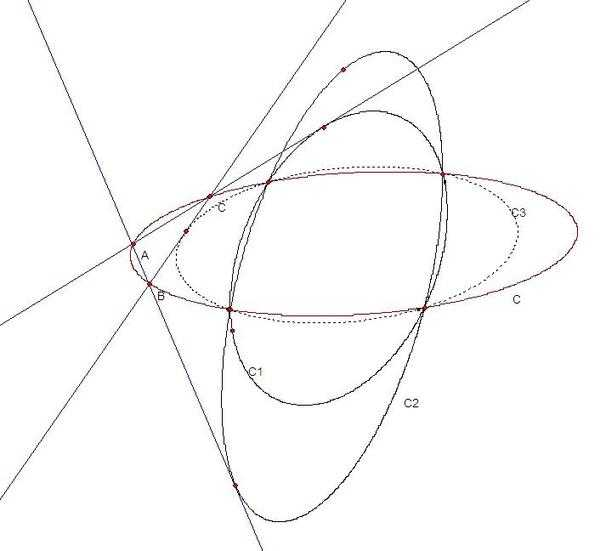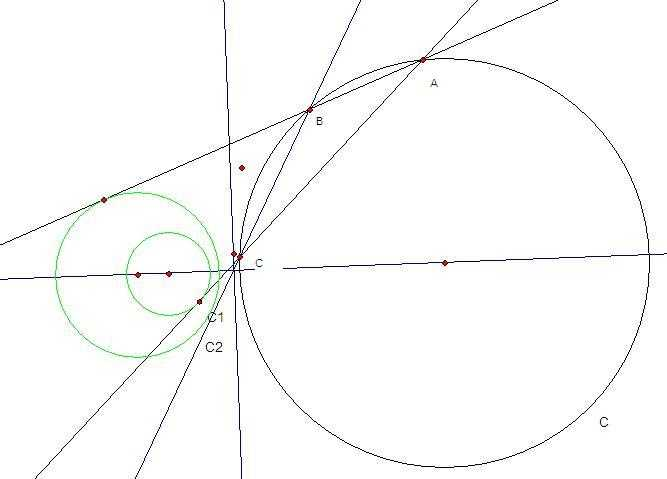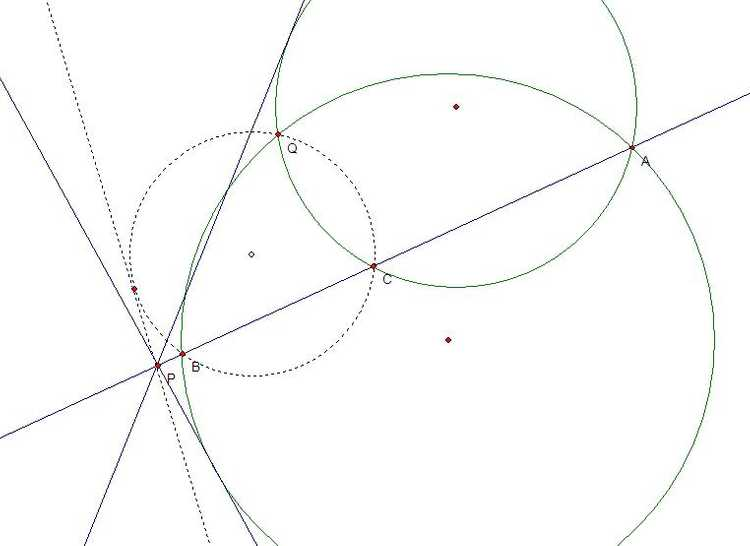$(x-a)(y-b)=(1-a)(1-b)$.

$\begin{bmatrix}x^2&x&1\end{bmatrix}\begin{bmatrix}b^2&-2b&1\\-2b&-2ab+4b+4a-2&-2a\\1&-2a&a^2\end{bmatrix}\begin{bmatrix}y^2\\y\\1\end{bmatrix}=0$.

$a^2b^2(st)^2-2ab(s+t)st+(s^2+t^2)+(-2ab+4a+4b-2)st-2(s+t)+1=0$

$\begin{bmatrix}s^2&s&1\end{bmatrix}\begin{bmatrix}a^2b^2&-2ab&1\\-2ab&-2ab+4a+4b-2&-2\\1&-2&1\end{bmatrix}\begin{bmatrix}t^2\\t\\1\end{bmatrix}=0$ (1)

## 简单推论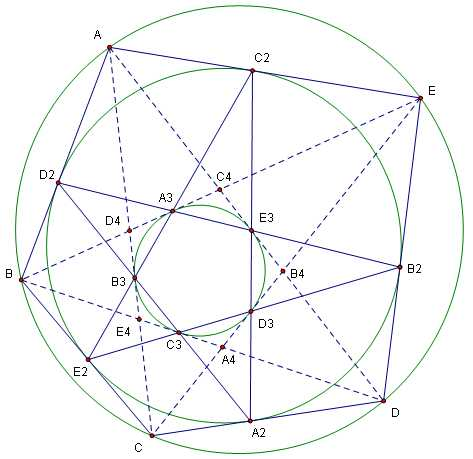## 各种逆定理

i)对于圆锥曲线C上一个二次对合变换T，连接C上任意一点P和像T(P)的直线同一个固定二次曲线相切
ii)对于圆锥曲线C上一个二次对合变换T,C上任意一点P和像T(P)处切线的交点在一个固定二次曲线上。
iii)对于圆锥曲线C上一个二次对合变换T,存在二次曲线$C_1$使得对于C上任意一点P,像T(P)在P关于$C_1$的极线上
iv)对于圆锥曲线C上一个二次对合变换T,存在二次曲线$C_1$使得对于C上任意一点P处切线l,像T(P)处切线过l关于$C_1$的极点。

v)对于直线l上任意一个二次对合变换T和一条同l相切的圆锥曲线C,过l上动点P和像T(P)的C的另外一条切线（不是l）的交点的轨迹是一条二次曲线
vi)对于过Q点的线束上的一个二次对合变换T和过Q点的圆锥曲线C,任意过Q点直线l和像T(l)同C交点的连线切一固定二次曲线。

## 特殊的退化的二次对合变换

i)恒等变换是特殊的二次对合变换，它是在圆锥曲线的第一类变换曲线同自身重合时的结果。它是唯一一个有三个以上恒等元的二次对合变换（恒等元是指这个元的两个像都是自身）
ii)对合变换也是特殊的二次对合变换，它是在圆锥曲线的第一类变换曲线退化为两条直线时的结果。它相当于过两条直线交点的任意直线同目标曲线两个交点之间确定的对合变换。对合变换中任何一个元的两个像都相同。而对于普通的二次对合变换，最多有四个元的两个像都相同，这些为反射点。同样对于普通的二次对合变换，如果某个元的两个像中有一个是自身，我们称这样的点为驻点，而对于普通的二次对合变换，最多有四个驻点。

## 射影变换的保二次对合变换性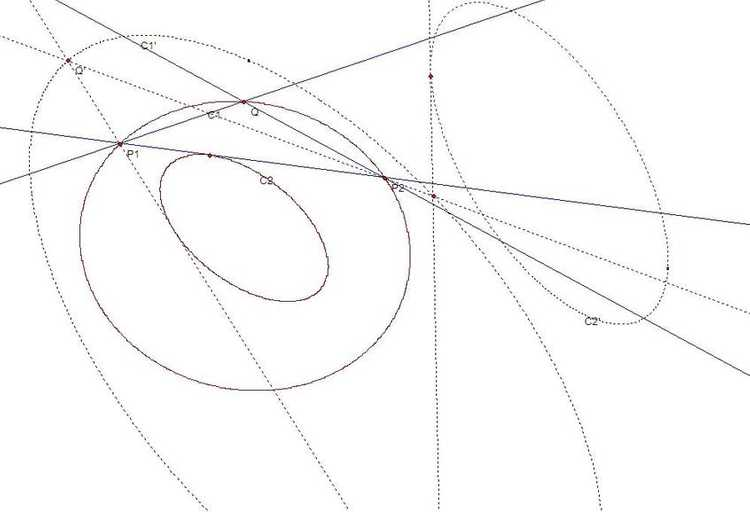# 二次对合变化与椭圆曲线群

## 射影变换和对合变换

$(x_1^2,x_1y_1,y_1^2 )C\begin{pmatrix}x_2^2\\x_2y_2\\y_2^2\end{pmatrix}=0$,我们称这是点$(x_1,y_1 )$到点$(x_2,y_2 )$的二次对合变换。

## 二次对合变换群中的运算

$(s^2,s,1)\begin{bmatrix}a^2 b^2&-2ab&1\\-2ab&-2ab+4a+4b-2&-2\\1&-2&1)\end{bmatrix}\begin{pmatrix}t^2\\t\\1\end{pmatrix}=0$

$(t^2,t,1)\begin{bmatrix}a^2 b^2&-2ab&1\\-2ab&-2ab+4a+4b-2&-2\\1&-2&1\end{bmatrix}\begin{pmatrix}1\\1\\1\end{pmatrix}=0$

$(x^2,x,1)\begin{bmatrix}a^2 b^2&-2ab&1\\-2ab&-2ab+4a+4b-2&-2\\1&-2&1\end{bmatrix}\begin{pmatrix}t_n^2\\t_n\\1\end{pmatrix}=0$

$\left({x}^{2},x,1\right)\left[\begin{array}{ccc}{a}^{2}{b}^{2}& -2ab& 1\\ -2ab& -2ab+4a+4b-2& -2\\ 1& -2& 1\end{array}\right]\left(\begin{array}{c}\end{array}$